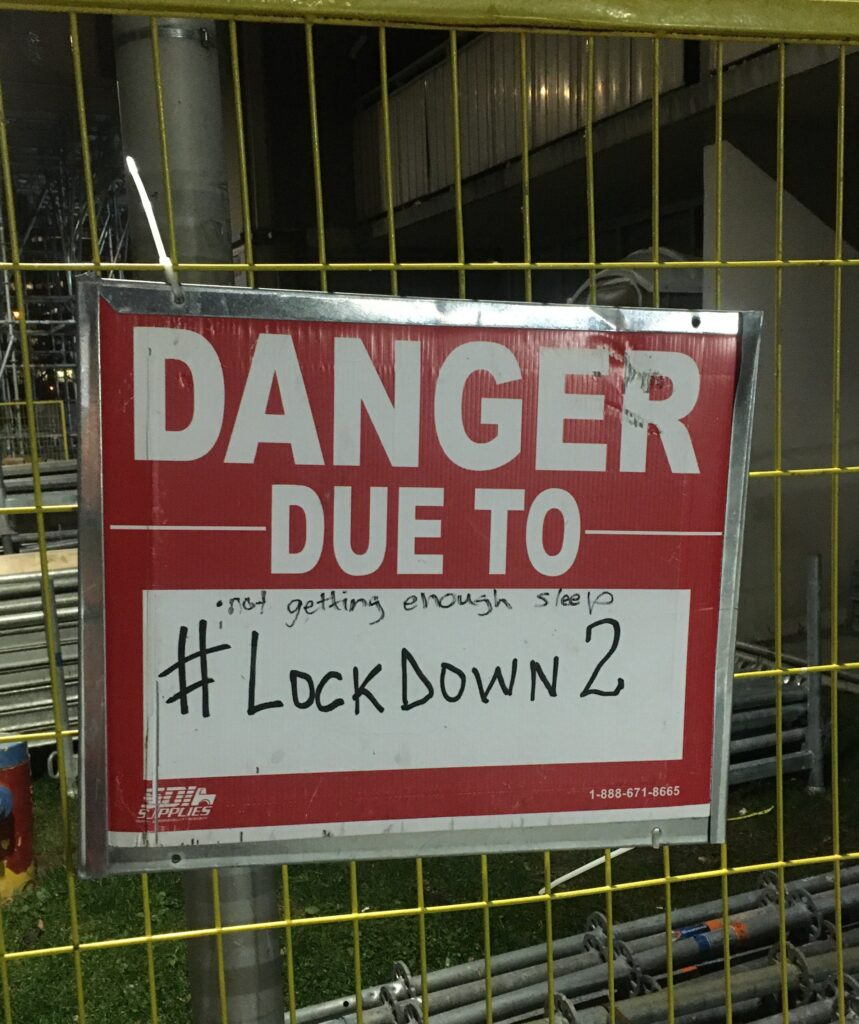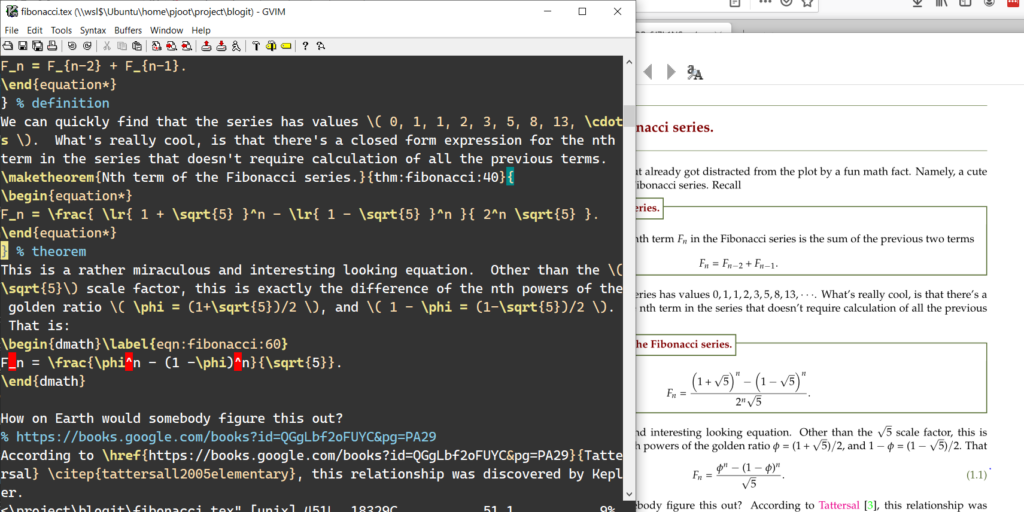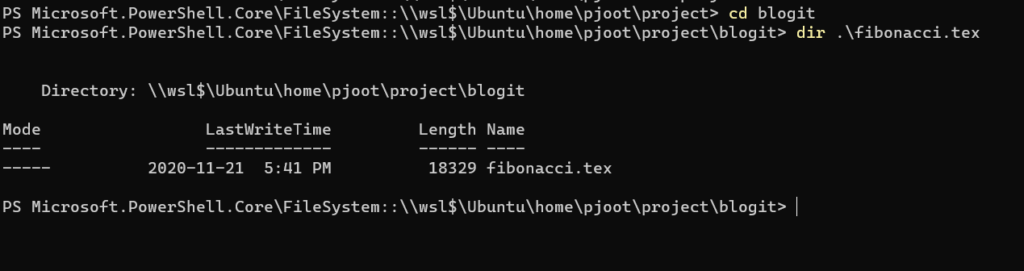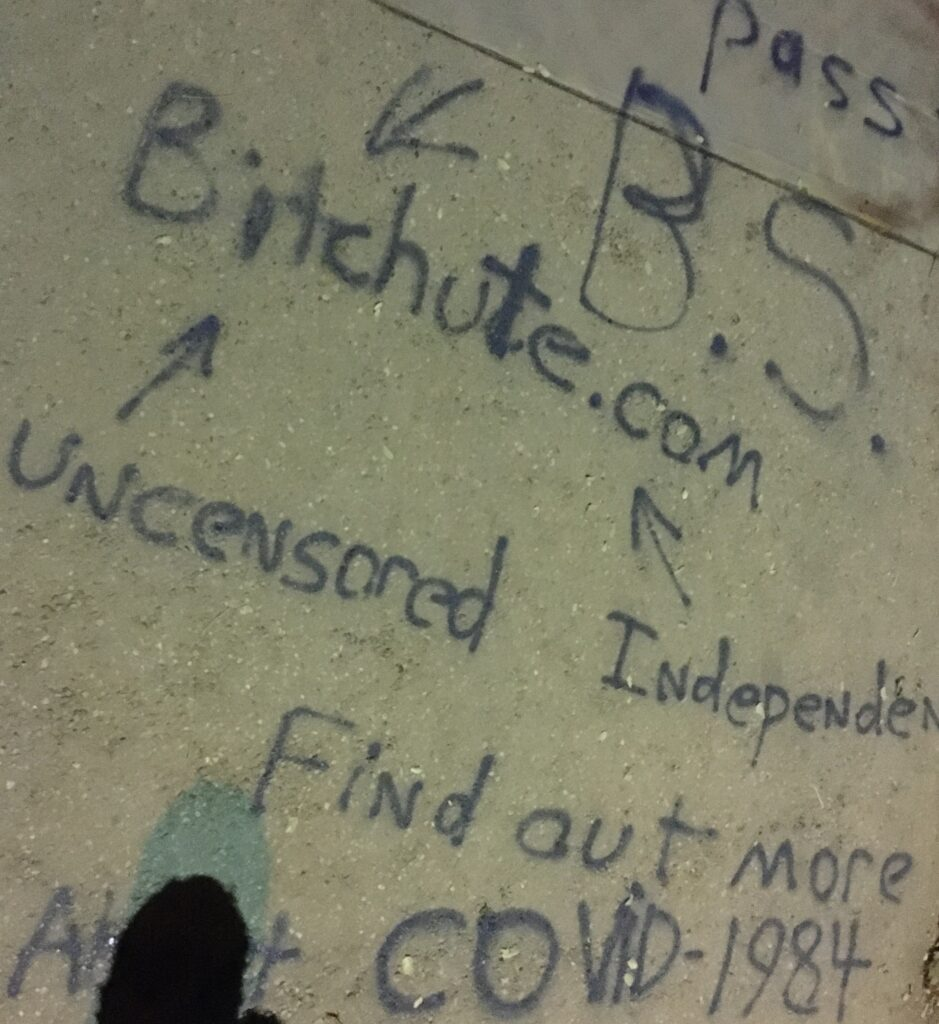## More anti-lockdown signage

November 28, 2020 Incoherent ramblings , ,

Not everybody wants to live in fear, have their jobs, savings and livelyhood taken away by zealous authoritarian tyrants that are desperate to appear as if they are doing something:I haven’t checked out the website above, but like the sentiment.  If you want to be driven to substance abuse, or live life with all privileges doled out to you like a slave, then maybe lockdowns are for you.

## Danger due to: Lockdown #2

November 27, 2020 Incoherent ramblings , , , ,

Somebody appears to have left a blank “Danger due to…” sign at a local construction site.There’s two captions, both apt. The second is also likely also a true harbinger of danger, since I’m sure not enough sleep is also a significant source of on the job injury and death.

## A minimally configured Windows laptop

November 22, 2020 Windows , , , , , ,

I’ve now installed enough that my new Windows machine is minimally functional (LaTex, Linux, and Mathematica), with enough installed that I can compile any of my latex based books, or standalone content for blog posts.  My list of installed extras includes:

• Brother HL-2170W (printer driver)
• Windows Terminal
• GPL Ghostscript (for MaTeX, latex labels in Mathematica figures.)
• Wolfram Mathematica
• Firefox
• Chrome
• Visual Studio
• Python
• Julia
• Discord
• OBS Studio
• MikTeX
• SumatraPDF
• GVim
• Git
• PowerShell (7)
• Ubuntu
• Dropbox

Some notes:

• On Windows, for my LaTeX work, I used to use MikTex + cygwin.  The cygwin dependency was for my makefile dependencies (gnu-make+perl).  With this new machine, I tried WSL2.  I’m running my bash shells within the new Windows Terminal, which is far superior to the old cmd.
• Putty is no longer required.  Windows Terminal does the job very nicely.  It does terminal emulation well enough that I can even ssh into a Linux machine and use screen within my Linux session, and my .screenrc just works.  Very nice.
• SumatraPDF is for latex reverse tex lookup.  i.e. I can double click on pdf content, and up pops the editor with the latex file.  Last time I used Sumatra, I had to configure it to use GVim (notepad used to be the default I think.)  Now it seems to be the default (to my suprise.)
• I will probably uninstall Git, as it seems superfluous given all the repos I want to access are cloned within my bash file system.
• I used to use GVim extensively on Windows, but most of my editing has been in vim in the bash shell.  I expect I’ll now only use it for reverse tex (–synctex) lookup editing.

WSL2 has very impressive integration.  A really nice demo of that was access of synctex lookup.  Here’s a screenshot that shows it in action:I invoked the windows pdf viewer within a bash shell in the Ubuntu VM, using the following:


pjoot@DESKTOP-6J7L1NS:~/project/blogit$alias pdfview alias pdfview='/mnt/c/Users/peete/AppData/Local/SumatraPDF/SumatraPDF.exe' pjoot@DESKTOP-6J7L1NS:~/project/blogit$ pdfview fibonacci.pdf


The Ubuntu filesystem directory has the fibonacci.synctex.gz reverse lookup index that Summatra is able to read. Note that this file, after unzipping, has only Linux paths (/home/pjoot/…), but Summatra is able to use those without any trouble, and pops up the (Windows executable) editor on the files after I double click on the file. This sequence is pretty convoluted:

• Linux bash ->
• invoke Windows pdf viewer ->
• that program reading Linux files ->
• it invokes a windows editor (presumably using the Linux path), and that editor magically knows the path to the Linux file that it has to edit.

Check out the very upper corner of that GVim window, where it shows the \\wsl\$\Ubuntu\home\pjoot\project\blogit\fibonacci.tex pathNot all applications know how to access files with UNC paths (for example, the old crappy cmd.exe cannot), but so far all the ones I have cared about have been able to do so.

## Public service announcement graffiti?

November 21, 2020 Incoherent ramblings , , ,

On Wednesday night’s Tessa walk, I encountered a very large piece of public service announcement graffiti:This is on a very wide pedestrian bridge, spanning the DVP in Riverdale.  I assume that bitchute is one of the uncensorable platforms like Dtube (a blockchain based video platform), but I haven’t actually checked it out.  I guess I’m not the only one that is annoyed that the big social media platforms have made it their policy to regulate the set of allowed opinions.  I wouldn’t have expressed myself with graffiti, but I guess that I’m not alone in my distaste for being told what I am allowed to think, or that some information is too dangerous for me to be allowed to look at it.

## Deriving the nth Fibonacci number formula.

[If mathjax doesn’t display properly for you, click here for a PDF of this post]

My last three posts:

were all about a cool formula for the n-th term of the Fibonacci series.  Here’s the final chapter of the story of that play.  A recap:

## Definition 1.1: Fibonacci series.

With $$F_0 = 0$$, and $$F_1 = 1$$, the nth term $$F_n$$ in the Fibonacci series is the sum of the previous two terms
\begin{equation*}
F_n = F_{n-2} + F_{n-1}.
\end{equation*}

## Theorem 1.1: Nth term of the Fibonacci series.

\begin{equation*}
F_n = \frac{ \lr{ 1 + \sqrt{5} }^n – \lr{ 1 – \sqrt{5} }^n }{ 2^n \sqrt{5} }.
\end{equation*}

## Deriving the nth Fibonacci formula.

There was a particularly unsatisfactory aspect of the guess that we made in the last post. In particular, we didn’t have any reason to guess the form of that solution, except for the fact that we already knew the answer. Now we will attempt to attack this in a more systematic fashion, so that each step along the way seems logical. First, we need to put a couple goodies in our toolbox.

## Definition 1.1: Discrete sum.

Given a set of discrete values $$\setlr{G_a, G_{a+1}, \cdots, G_n }$$ we define a discrete sum of $$n – a + 1$$ of these terms as $$F_n$$
\begin{equation}\label{eqn:fibonacciblog:1540}
F_n = \sum_{k = a}^n G_k + C,
\end{equation}
where $$C$$ is an arbitrary boundary value constant.

## Definition 1.2: Difference operators.

Define a backwards difference operator $$\Delta$$, operating on $$X_n$$ as
\begin{equation}\label{eqn:fibonacciblog:1560}
\Delta X_n = X_n – X_{n-1}.
\end{equation}

The difference operator is a discrete analogue of a differential operator. It is also possible to define a (forward) difference operator as $$\Delta X_n = X_{n+1} – X_{n}$$, but the choice is arbitary, and we can find the same results either way.

## Lemma 1.1: Antidifference of discrete sum.

Given a sum $$F_n$$ of the form \ref{eqn:fibonacciblog:1540}, the difference operation is just the highest $$n$$ term of the sum
\begin{equation}\label{eqn:fibonacciblog:1580}
\Delta F_n = G_n.
\end{equation}

### Start proof:

\begin{equation}\label{eqn:fibonacci:960}
\Delta F_n =
\sum_{k = a}^n G_k + C
– \lr{ \sum_{k = a}^{n-1} G_k + C }
= G_n.
\end{equation}

### End proof.

Computing differences is pretty easy. What we want to do is the inverse operation (analogous to integration), where we find a closed form representation of $$F_n$$ given a difference equation $$\Delta F_n = G_n$$. Just as we can compute antiderivatives for $$x^n$$, we may do the same for $$n^k$$ antidifferences, but the results are messier. The first few such antidifferences are

## Theorem 1.1: Antidifferences for powers of $$n$$.

\begin{equation}\label{eqn:fibonacciblog:1600}
\begin{aligned}
1 &= \Delta n \\
n &= \Delta \lr{ \frac{n}{2}\lr{ n + 1} } \\
n^2 &= \Delta \lr{ \frac{n}{6}\lr{2 n + 1}\lr{n + 1} } \\
n^3 &= \Delta \lr{ \frac{n^2}{4}\lr{n + 1}^2 }.
\end{aligned}
\end{equation}

### Start proof:

The $$\Delta n$$ identity is easily verified
\begin{equation}\label{eqn:fibonacci:1000}
\Delta n = n – (n-1) = 1.
\end{equation}
For higher orders it is a bit tedious to verify directly, but we can iteratively build up those results by evaluating the difference operator on each of the powers of $$n$$.
\begin{equation}\label{eqn:fibonacci:660}
\begin{aligned}
\Delta n^2
&= n^2 – (n-1)^2 \\
&= n^2 – (n^2 – 2 n + 1) \\
&= 2 n – 1, \\
&= 2 n – \Delta n.
\end{aligned}
\end{equation}
Because the difference operator is linear, we can rearrange to find
\begin{equation}\label{eqn:fibonacci:1020}
\Delta \lr{ n^2 + n } = 2 n.
\end{equation}
Dividing through by $$2$$ and factoring out an $$n$$, recovers the desired result.

For the next power, we have
\begin{equation}\label{eqn:fibonacci:680}
\begin{aligned}
\Delta n^3
&= n^3 – (n-1)^3 \\
&= n^3 – (n^3 – 3 n^2 + 3 n – 1) \\
&= 3 n^2 – 3 n + 1 \\
&= 3 n^2 – 3 \Delta \frac{n}{2}\lr{ n + 1 } + \Delta n,
\end{aligned}
\end{equation}
or
\begin{equation}\label{eqn:fibonacci:1040}
\begin{aligned}
3 n^2
&=
\Delta \lr{n} \lr{ n^2 + \frac{3}{2}\lr{ n + 1} – 1 } \\
&=
\Delta \frac{n}{2} \lr{ 2 n^2 + 3\lr{ n + 1} – 2} \\
&=
\Delta \frac{n}{2} \lr{ 2 n^2 + 3 n + 1 } \\
&=
\Delta \frac{n}{2} \lr{ 2 n + 1}\lr{ n + 1 }
\end{aligned}
\end{equation}
Dividing through by $$3$$ recovers the desired result.

The final result is left to the reader. It can be derived or verified easily with a couple lines of Mathematica code.

## Problem: Sum some series.

Find the sums $$\sum_{k = 1}^n k^m$$, for $$m = 1, 2, 3$$.

• $$m = 1$$. This is the (probably apocryphal) sum of Gauss’s grade school classroom:
\begin{equation}\label{eqn:fibonacci:1060}
F_n = \sum_{k = 1}^n k = 1 + 2 + \cdots n,
\end{equation}
satisfying
\begin{equation}\label{eqn:fibonacci:1080}
\begin{aligned}
\Delta F_n
&= F_n – F_{n-1} \\
&=
(n + (n-1) + \cdots + 1)

((n-1) + \cdots + 1) \\
&= n \\
&= \Delta \frac{n}{2}(n + 1).
\end{aligned}
\end{equation}
We must have
\begin{equation}\label{eqn:fibonacci:1100}
F_n = \frac{n}{2}\lr{ n + 1} + C.
\end{equation}
To fix $$C$$ consider $$F_1$$
\begin{equation}\label{eqn:fibonacci:1180}
F_1 = \inv{2}(1 + 1) + C = 1,
\end{equation}
so $$C = 0$$, so we find Gauss’s summation formula
\begin{equation}\label{eqn:fibonacci:1200}
\sum_{k = 1}^n k = \frac{n}{2}\lr{ n + 1},
\end{equation}
as expected.
• $$m = 2$$. Now let’s do the sum of squares
\begin{equation}\label{eqn:fibonacci:1120}
F_n = \sum_{k = 1}^n k^2,
\end{equation}
for which we have
\begin{equation}\label{eqn:fibonacci:1140}
\Delta F_n = n^2 = \Delta \frac{n}{6}( 2 n + 1 )(n+1),
\end{equation}
so
\begin{equation}\label{eqn:fibonacci:1160}
F_n = \frac{n}{6}( 2 n + 1 )(n+1) + C.
\end{equation}
Clearly $$C = 0$$ satisfies the boundary condition, leaving
\begin{equation}\label{eqn:fibonacci:1220}
\sum_{k = 1}^n k^2 =
\frac{n}{6}( 2 n + 1 )(n+1).
\end{equation}
• $$m = 3$$. We see the pattern, so for the sum of cubes, we can just write down the answer
\begin{equation}\label{eqn:fibonacci:1240}
\sum_{k = 1}^n k^3 =
\frac{n^2}{4}\lr{n + 1}^2
.
\end{equation}

Now that we have some basic comfort with the ideas of difference equations, and their solutions,
let’s get back to the Fibonacci problem. In that case, we have
\begin{equation}\label{eqn:fibonacci:1260}
F_n = F_{n-1} + F_{n-2}.
\end{equation}
Stated as a difference equation, this is
\begin{equation}\label{eqn:fibonacci:1280}
\Delta F_n = F_{n-2}.
\end{equation}
Before tackling the Fibonacci problem, let’s try one that slightly simpler.

## Problem: A simpler problem.

Solve $$\Delta F_n = F_{n-1}$$, where $$F_0 = 0, F_1 = 1$$.

The problem to solve is just
\begin{equation}\label{eqn:fibonacci:1300}
F_n = 2 F_{n-1}.
\end{equation}
This sequence is $$\setlr{ 1, 2, 4, 8, \cdots }$$, so we can solve it by inspection, and the answer is just $$F_n = 2^{n-1}$$. We want inspiration for the Fibonacci problem, so let’s pretend that we can’t see the answer, but that we can guess something close, and see if it works. Namely, let’s guess:
\begin{equation}\label{eqn:fibonacci:1320}
F_n = \alpha a^n + C.
\end{equation}
If we plug this trial solution into our difference equation, we get
\begin{equation}\label{eqn:fibonacci:1340}
\begin{aligned}
\alpha a^{n-1} + C
&=
\Delta F_n \\
&= \alpha \lr{ a^n – a^{n-1} } \\
&= \alpha a^{n-1} \lr{ a – 1 }
\end{aligned}
\end{equation}
This can be satisfied by setting $$C = 0$$ and $$a – 1 = 1$$, or $$a = 2$$, as we already knew. To fix the constant $$\alpha$$ we utilize our boundary constraints, namely
\begin{equation}\label{eqn:fibonacci:1400}
F_1 = 1 = \alpha 2
\end{equation}
so $$\alpha = 1/2$$.

Compared to just seeing the answer, the procedure above was a lot of work. However, a side effect of this work is discovery of a guessing strategy that is somewhat like using $$f(t) = e^{s t}$$ to generate a characteristic equation when solving a differential equation. For a difference equation of this form, it appears we can substitute $$F_n = \alpha a^n + C$$ and use the differences to determine the values of $$\alpha, a, C$$. Now let’s try this with the Fibonacci difference equation.

## Problem: Find a solution to the Fibonacci difference equation.

Without worrying about boundary constraints, find the solutions to $$\Delta F_n = F_{n-2}$$, using a trial solution of $$F_n = \alpha a^n$$.

Inserting our trial solution, we have
\begin{equation}\label{eqn:fibonacci:1420}
\begin{aligned}
\alpha a^n
&=
F_n \\
&= F_{n-1} + F_{n-2} \\
&= \alpha \lr{ a^{n-1} + a^{n-2} } \\
&= \alpha a^{n-2} \lr{ a + 1 },
\end{aligned}
\end{equation}
so our “characteristic equation” is
\begin{equation}\label{eqn:fibonacci:1440}
a + 1 = a^2.
\end{equation}
Completing the square yields
\begin{equation}\label{eqn:fibonacci:1460}
\lr{ a – \inv{2} }^2 = 1 + \inv{4},
\end{equation}
or
\begin{equation}\label{eqn:fibonacci:1480}
a = \inv{2} \pm \frac{\sqrt{5}}{2}.
\end{equation}
Bamn. There’s our golden ratio, and it’s buddy!
We find that
\begin{equation}\label{eqn:fibonacci:1500}
F_n = \alpha \lr{\frac{1 \pm \sqrt{5}}{2} }^n,
\end{equation}
are solutions to the difference equation \ref{eqn:fibonacci:1280}.

Since we have a second order difference equation, we need a superposition of both solutions to try to satisfy the boundary conditions. In particular, we want to find the constants
\begin{equation}\label{eqn:fibonacci:1520}
F_n =
\alpha_{+} \lr{\frac{1 + \sqrt{5}}{2} }^n
+
\alpha_{-} \lr{\frac{1 – \sqrt{5}}{2} }^n + C.
\end{equation}
However, we already did this when we guessed used $$F_n = \alpha a^n + \beta b^n$$ as a trial solution. When we did that, it was just to see if we could find the end result, knowing only the structure of the solution, but none of the specific constants. Now we have justified why that was a reasonable trial solution, since exactly this structure follows naturally from the difference equation itself.

This train of thought, makes me want to dig out my little Dover book on difference equations  that I’ve had since I was a kid. I think I only worked through the first chapter of that book. I have a lot of little sad neglected Dover books on mathematics and physics that I bought super cheap at the World’s Biggest Bookstore when I was back in school. It will be interesting to see how to tackle problems such as this, in a still more systematic fashion.

# References

 Hyman Levy and Freda Lessman. Finite difference equations. Courier Corporation, 1992.